SSC BOARD PAPERS IMPORTANT TOPICS COVERED FOR BOARD EXAM 2024

### Problem Set 3 Circle Class 10th Mathematics Part 2 MHB Solution

Problem Set 3

1. Two circles of radii 5.5 cm and 3.3 cm respectively touch each other. What is the…
2. Two circles intersect each other such that each circle passes through the centre of…
3. A circle touches all sides of a parallelogram. So the parallelogram must be…
4. Length of a tangent segment drawn from a point which is at a distance 12.5 cm from the…
5. If two circles are touching externally, how many common tangents of them can be drawn?…
6. ∠ ACB is inscribed in arc ACB of a circle with centre O. If ∠ ACB = 65°,find m(arc…
7. Chords AB and CD of a circle intersect inside the circle at point E. If AE = 5.6,EB =…
8. In a cyclic square ABCD, twice the measure of angle a is thrice the measure of…
9. Points A, B, C are on a circle, such that m(arc AB) = m(arc BC) = 120°. Nopoint,…
10. Seg XZ is a diameter of a circle. Point Y lies in its interior. How many of the…
11. Line l touches a circle with centre Oat point P. If radius of the circle is 9 cm,answer…
12. In figure 3.83, M is the centre of the circle and seg KL is a tangent segment.If MK =…
13. In figure 3.84, O is the centre of the circle. Seg AB, seg AC are tangent segments.…
14. In figure 3.85, square aboc is a parallelogram. It circumscribes the circle with…
15. In figure 3.86, circle with centre M touches the circle with centre N at point T.…
16. In the adjoining figure circles with centres X and Y touch each other at point Z. A…
17. In figure 3.88, circles with centres X and Y touch internally at point Z. Seg BZ is a…
18. In figure 3.89, line l touches the circle with centre O at point P. Q is the mid point…
19. In figure 3.90, seg AB is a diameter of a circle with centre C. Line PQ is a tangent,…
20. Draw circles with centres A, B and C each of radius 3 cm, such that each circle…
21. Prove that any three points on a circle cannot be collinear.
22. In figure 3.91, line PR touches the circle at point Q. Answer the following questions…
23. In figure 3.92, O is the centre of a circle, chord PQ ≅ chord RS If∠ POR = 70°and (arc…
24. In figure 3.93, m(arc WY) = 44°,m(arc ZX) = 68°, then(1) Find the measure of ∠ ZTX.(2)…
25. In figure 3.94,(1) m(arc CE) = 54°,m(arc BD) = 23°, find measure of ∠CAE.(2) If AB =…
26. In figure 3.95, chord EF || chord GH. Prove that, chord EG ≅ chord FH.Fill in the…
27. In figure 3.96 P is the point of contact.(1) If m(arc PR) = 140°,∠ POR = 36°,find…
28. In figure 3.97, circles with centres C and D touch internally at point E. D lies on…
29. In figure 3.98, seg AB is a diameter of a circle with centre O . The bisector of∠ ACB…
30. In figure 3.99, seg MN is a chord of a circle with centre O. MN = 25,L is a point on…
31. In figure 3.100, two circles intersect each other at points S and R. Their common…
32. In figure 3.101, two circles intersect at points M and N. Secants drawn through M and…
33. In figure 3.102, two circles intersect each other at points A and E. Their common…
34. In figure 3.103, seg AD side BC, seg BE side AC, seg CF side AB. Point O is the…

###### Problem Set 3
Question 1.

Four alternative answers for each of the following questions are given. Choose the correct alternative.

Two circles of radii 5.5 cm and 3.3 cm respectively touch each other. What is the distance between their centers?

A. 4.4 cm

B. 8.8 cm

C. 2.2 cm

D. 8.8 or 2.2 cm

Given that both the circles touch each other but not specified externally or internally.

The distance between the centres of the circles touching internally is equal to the difference of their radii.

⇒distance between their centres = 5.5 cm – 3.3 cm = 2.2 cm

If the circles touch each other externally, distance between their centres is equal to the sum of their radii.

⇒distance between their centres = 5.5 cm + 3.3 cm = 8.8 cm

Question 2.

Four alternative answers for each of the following questions are given. Choose the correct alternative.

Two circles intersect each other such that each circle passes through the centre of the other. If the distance between their centres is 12, what is the radius of each circle?

A. 6 cm

B. 12 cm

C. 24 cm

D. can’t say

Given OA = 12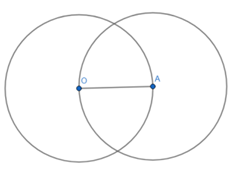From the figure, OA is the radius of both the circles.

Given that distance between their centres is OA = 12

∴ Radius of the circles = 12

Question 3.

Four alternative answers for each of the following questions are given. Choose the correct alternative.

A circle touches all sides of a parallelogram. So the parallelogram must be a,................... .

A. rectangle

B. rhombus

C. square

D. trapezium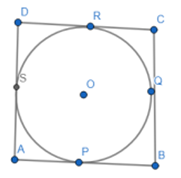Let ABCD be a parallelogram which circumscribes the circle.

AP = AS [Tangents drawn from an external point to a circle are equal in length]

BP = BQ [Tangents drawn from an external point to a circle are equal in length]

CR = CQ [Tangents drawn from an external point to a circle are equal in length]

DR = DS [Tangents drawn from an external point to a circle are equal in length]

Consider, (AP + BP) + (CR + DR) = (AS + DS) + (BQ + CQ)

AB + CD = AD + BC

But AB = CD and BC = AD [Opposite sides of parallelogram ABCD]

AB + CD = AD + BC

Hence 2AB = 2BC

Therefore, AB = BC

Similarly, we get AB = DA and DA = CD

Thus, ABCD is a rhombus.

Question 4.

Four alternative answers for each of the following questions are given. Choose the correct alternative.

Length of a tangent segment drawn from a point which is at a distance 12.5 cm from the centre of a circle is 12 cm, find the diameter of the circle.

A. 25 cm

B. 24 cm

C. 7 cm

D. 14 cm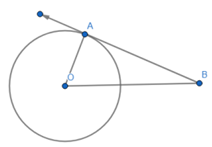Given: BO = 12.5 cm and AB = 12cm

In ∆AOB,

∠OAB = 90° {Using tangent-radius theorem which states that a tangent at any point of a circle is perpendicular to the radius at the point of contact.}

BO2 = AB2 + OA2 {Using Pythagoras theorem}

⇒ (12.5)2 = 122 + OA2

⇒ OA2 = 156.25 - 144

⇒ OA = √12.25

⇒ OA = 3.5cm

⇒ Diameter = 7 cm

Question 5.

Four alternative answers for each of the following questions are given. Choose the correct alternative.
If two circles are touching externally, how many common tangents of them can be drawn?
A. One

B. Two

C. Three

D. Four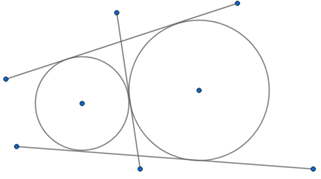If two circles are touching each other externally, they have 3 tangents in common. The above figure proves this statement.

There are three common tangets for the given two circles.

Question 6.

Four alternative answers for each of the following questions are given. Choose the correct alternative.

∠ ACB is inscribed in arc ACB of a circle with centre O. If ∠ ACB = 65°,find m(arc ACB).

A. 65°

B. 130°

C. 295°

D. 230°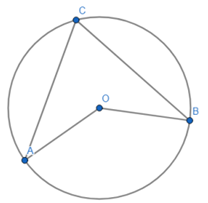Given ∠ACB = 65°

⇒∠ AOB = 2 × 65° = 130° {∵ The measure of an inscribed angle is half the measure of the arc intercepted by it.}

m(AB) = 130°

So, m(arc ACB ) = 360° - m(AB) {∵ Measure of a major arc = 360°- measure of its corresponding minor arc}

⇒m(arc ACB ) = 360° - 130° = 230°

Question 7.

Four alternative answers for each of the following questions are given. Choose the correct alternative.

Chords AB and CD of a circle intersect inside the circle at point E. If AE = 5.6,EB = 10, CE = 8, find ED.

A. 7

B. 8

C. 11.2

D. 9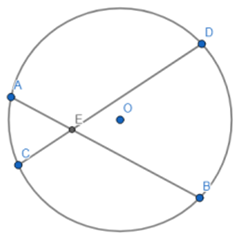Given: AE = 5.6,EB = 10, CE = 8

We know that AE × EB = CE × ED

This property is known as theorem of chords intersecting inside the circle.

⇒ 5.6 × 10 = 8 × ED

⇒ ED = 7

Question 8.

Four alternative answers for each of the following questions are given. Choose the correct alternative.

In a cyclic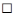ABCD, twice the measure of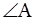is thrice the measure of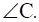Find the measure of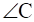?

A. 36

B. 72

C. 90

D. 108

Given that 2∠ A = 3∠ C

We know that in a cyclic quadrilateral opposite angles are supplementary to each other.

⇒∠ A + ∠ C = 180°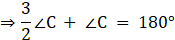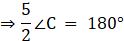⇒∠ C = 72°

Question 9.

Four alternative answers for each of the following questions are given. Choose the correct alternative.

Points A, B, C are on a circle, such that m(arc AB) = m(arc BC) = 120°. Nopoint, except point B, is common to the arcs. Which is the type of ∆ ABC?

A. Equilateral triangle

B. Scalene triangle

C. Right angled triangle

D. Isosceles triangle

Angle subtended by the arcs at centre = 120°

⇒ Angle subtended by the arc at the remaining part of the circle = 60° {The measure of an inscribed angle is half the measure of the arc intercepted by it.}

∵ Interior angles of the triangle ABC = 60°

∴It is an equilateral triangle.

Question 10.

Four alternative answers for each of the following questions are given. Choose the correct alternative.

Seg XZ is a diameter of a circle. Point Y lies in its interior. How many of the following statements are true?

(i) It is not possible that ∠XYZ is an acute angle.

(ii) ∠ XYZ can’t be a right angle.

(iii) ∠ XYZ is an obtuse angle.

(iv) Can’t make a definite statement for measure of ∠ XYZ.

A. Only one

B. Only two

C. Only three

D. All

If Y would have lied on circumference ∠ XYZ = 90° since XZ is the diameter.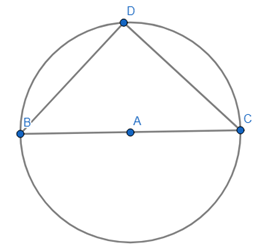If Y lied outside the circle, ∠ XYZ = acute angle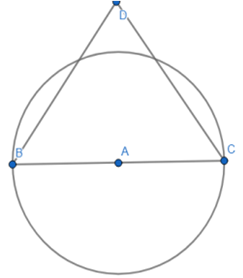∴∠ XYZ is an obtuse angle.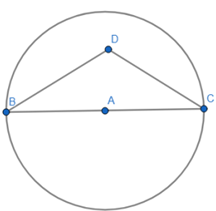Statements (i), (ii) and (iii) are true.

Question 11.

Line l touches a circle with centre Oat point P. If radius of the circle is 9 cm,answer the following.

(1) What is d(O, P) = ? Why ?

(2) If d(O, Q) = 8 cm, where does thepoint Q lie?

(3) If d(PQ) = 15 cm, How manylocations of point R are line online l ? At what distance will eachof them be from point P?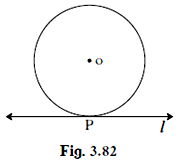(1)The perpendicular distance of O from P = radius of the circle = 9 cm.

(2)Q lies in the interior of the circle because P lieing on the circumference of the circle is at a distance of 9 cm.

(3)Position of R is not specified.

Question 12.

In figure 3.83, M is the centre of the circle and seg KL is a tangent segment.

If MK = 12, KL =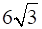then find -

(2) Measures of ∠ K and ∠ M.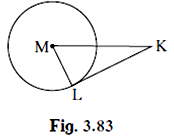(1) Here LM is the radius of the circle

⇒ ∠KML = 90° Using tangent-radius theorem which states that a tangent at any point of a circle is perpendicular to the radius at the point of contact.

In triangle MLK right-angled at L,

Given MK = 12, KL = 6√3,

MK2 = LM2 + KL2 {Using Pythagoras theorem}

⇒LM2 = 122 -6√32

⇒LM2 = 144 - 108

⇒ LM = √36

⇒ LM = 6 cm

(2)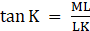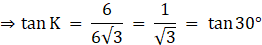⇒∠K = 30°

∠ M + ∠ K + ∠ L = 180° {Angle sum property of the triangle}

So, ∠ M = 180° - ∠ K - ∠ L

⇒ ∠ M = 180° - 30° - 90° = 60°

Question 13.

In figure 3.84, O is the centre of the circle. Seg AB, seg AC are tangent segments. Radius of the circle isr and l(AB) = r , Prove that,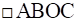is a square.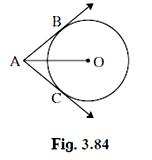Given: AB = r = radius of the circle

Here, AB = AC = r {tangents from the same external point are equal}

And OB = OC = r = radius of the circle.

⇒ ∠ OBA = ∠ OCA = 90° Using tangent-radius theorem which states that a tangent at any point of a circle is perpendicular to the radius at the point of contact.

∵ sides of ABOC are equal and opposite angles are 90° each

Hence, ABOC is a square.

Question 14.

In figure 3.85,is a parallelogram. It circumscribes the circle with cnetre T. Point E,F, G, H are touching points. If AE = 4.5,EB = 5.5, find AD.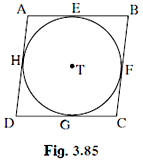Given: AE = 4.5, EB = 5.5

Here, AE = AH = 4.5 {tangents from same external point are equal}

EB = BF = 5.5{tangents from same external point are equal}

∵ Opposite sides of a parallelogram are equal

∴ AE = DG and EB = GC

Also, DH = DG = 4.5{tangents from same external point are equal}

And FC = GC = 5.5 {tangents from same external point are equal}

⇒ AD = AH + HD = 10

Question 15.

In figure 3.86, circle with centre M touches the circle with centre N at point T. Radius RM touches the smaller circle at S. Radii of circles are 9 cm and 2.5 cm. Find the answers to the following questions hence find the ratio MS:SR.

(1) Find the length of segment MT

(2) Find the length of seg MN

(3) Find the measure of ∠ NSM.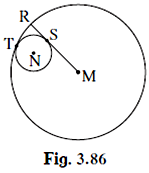(1)MT = radius of the big circle = 9 cm

(2)MN = MT – TN = 9 – 2.5 = 6.5 cm

(3)SM is the tangent to the circle with radius 2.5 cm with S being point of contact.

∠ NSM = 90° Using tangent-radius theorem which states that a tangent at any point of a circle is perpendicular to the radius at the point of contact.

In ∆MSN,

∠ MSN = 90°{∵ MS is the tangent to the small circle with point of contact S}

⇒ MN2 = MS2 + NS2

MS2 = MN2 – NS2

⇒ MS2 = 6.52 – 2.52

⇒ MS2 = 36

⇒ MS = 6 cm

Now, SR = MR – MS = 9 – 6 = 3 cm

⇒ MS:SR = 6:3 = 2:1

Question 16.

In the adjoining figure circles with centres X and Y touch each other at point Z. A secant passing through Z intersects the circles at points A and B respectively. Prove that, radius XA || radius YB. Fill in the blanks and complete the proof.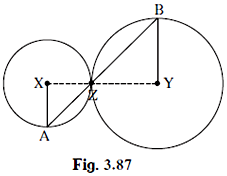Construction: Draw segments XZ and ..YZ........ .

Proof: By theorem of touching circles, points X, Z, Y are ..concyclic........ .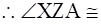..∠ YZB ........vertically opposite angles

Let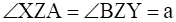..... (I)

Now, seg XA ≅seg XZ ........ (...radius of the same circle.......)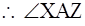= ....∠ XZA...... = a ........ (isosceles triangle theorem) (II)

similarly, seg YB ≅ .YZ......... ........ (.radius of the same circle.........)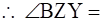.∠ZBY......... = a ........ (.isosceles triangle theorem.........) (III)

∴from (I), (II), (III),

∠ XAZ = .∠ ZBY.........

∴radius XA || radius YB .......... (..since alternate interior angles are equal........)

Construction: Draw segments XZ and YZ.

Proof: By theorem of touching circles, points X, Z, Y are concyclic.

XZA = ∠ YZB {vertically opposite angles}

Let ∠ XZA = ∠ BZY = a (I)

Now, seg XA ≅segXZ (radius of the same circle)

XAZ = ∠ XZA = a (isosceles triangle theorem) (II)

Similarly, seg YB ≅ YZ (radius of the same circle)

∴ ∠ BZY = ∠ZBY = a (isosceles triangle theorem) (III)

∴from (I), (II), (III),

∠ XAZ = ∠ ZBY

Question 17.

In figure 3.88, circles with centres X and Y touch internally at point Z. Seg BZ is a chord of bigger circle and intersects smaller circle at point A. Prove that, seg AX || seg BY.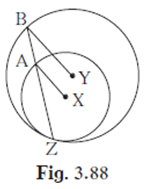XA and YB are the radii of the respective circles.

AZ and BZ are the chords of the circles.

In triangle XAZ,

AX = XZ {Radii of the same circle}

⇒ ∠ XAZ = ∠ XZA {angles opposite to equal sides are equal}

In triangle YBZ,

YB = YZ {Radii of the same circle}

⇒ ∠ YBZ = ∠ YZB {angles opposite to equal sides are equal}

⇒ ∠ XAZ = ∠ XZA = ∠ YBZ = ∠ YZB

∵ Corresponding angles are equal

⇒ XA||YB

Question 18.

In figure 3.89, line l touches the circle with centre O at point P. Q is the mid point of radius OP. RS is a chord through Q such that chords RS || line l. If RS = 12 find the radius of the circle.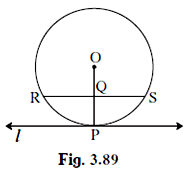The radius of the circle will bisect the chord RS. Therefore, RQ = QS = 1/2 × 12 = 6

Let the radius of circle be r,

Now, in Δ OQS, we have,

RQ = 6

OR = r

OQ = 1/2 r

Applying Pythagoras theorem, we get,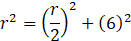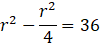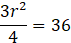3r2 = 4 × 36

r2 = 4 × 12 = 48

r = √48 units

Question 19.

In figure 3.90, seg AB is a diameter of a circle with centre C. Line PQ is a tangent, which touches the circle at point T. seg AP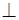line PQ and seg BQline PQ. Prove that, seg CP ≅seg CQ.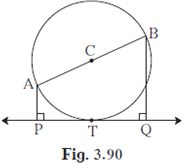To Prove: seg CP ≅seg CQ

Construction: Join CP, CQ and CT

Figure: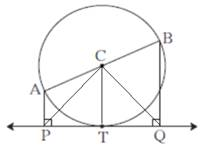Since PQ is a tangent to the circle, ∠CTP = ∠CTQ = 90

Since ∠APT = ∠CTP = 90

AP || CT.

Similarly,

CT || BQ.

So, we can say that,

AP || CT || BQ

AB is a line cutting all three parallel lines.

AC = CB (Radius of the circle, and AB is diameter, C is center)

Since C is center point of line AB cutting parallel lines.

We can say these parallel lines are equal distance.

Therefore, PT = TQ.

Now in ΔCTP and ΔCTQ,

CT is a common side, PT = TQ and ∠CTP = ∠CTQ = 90

CP = CQ. (Pythagoras theorem or congruent triangle theorem)

Hence, Proved.

Question 20.

Draw circles with centres A, B and C each of radius 3 cm, such that each circle touches the other two circles.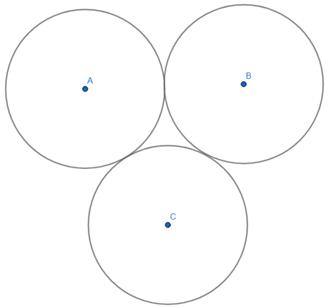Draw a circle with radius 3 and centred at A. Similarly draw other two circles with centre B and C and same radius touching each other externally.

Question 21.

Prove that any three points on a circle cannot be collinear.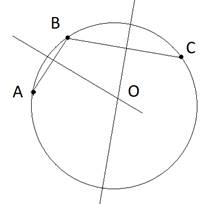We draw a circle of any radius and take any three points A, B and C on the circle.

We join A to B and B to C.

We draw perpendicular bisectors of AB and BC.

We know that perpendicular from the center bisects the chord.

Hence the center lies on both of the perpendicular bisectors.

The point where they intersect is the center of the circle.

The perpendiculars of the line segments drawn by joining collinear points is always parallel whereas in circle any three point’s perpendicular bisector will always intersect at the center.

Hence, any three points on the circle cannot be collinear.

Question 22.

In figure 3.91, line PR touches the circle at point Q. Answer the following questions with the help of the figure.

(1) What is the sum of ∠ TAQ and∠ TSQ?

(2) Find the angles which are congruent to ∠ AQP.

(3) Which angles are congruent to ∠QTS ?

(4) ∠ TAS = 65°, find the measure of ∠TQS and arc TS.

(5) If ∠AQP = 42°and ∠SQR = 58° find measure of ∠ATS.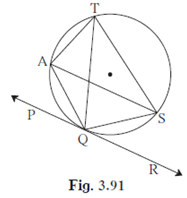(1) As TAQS is a cyclic quadrilateral,

∠TAQ + ∠TSQ = 180° (Sum of opposite angles of a cyclic quadrilateral is 180° )

(2) ∠ASQ and ∠ATQ

(3) ∠ QAS and ∠SQR

(4) ∠TAS = 65°

∠ TQS = ∠ TAS = 65° (angle by same arc TS in the same sector)

m(arc TS) = ∠TQS + ∠TAS

⇒ m(arc TS) = 65 + 65 = 130°

(5) ∠AQP + ∠AQS + ∠SQR = 180°

⇒ 42 + ∠AQS + 58 = 180

⇒ ∠AQS + 100 = 180

⇒ ∠AQS = 80

∠ AQS + ∠ ATS = 180° (opposite angles of a cyclic quadrilateral)

⇒ 80 + ∠ATS = 180

⇒ ∠ATS = 100°

Question 23.

In figure 3.92, O is the centre of a circle, chord PQ ≅ chord RS If∠ POR = 70°and (arc RS) = 80°, find -

(1) m(arc PR)

(2) m(arc QS)

(3) m(arc QSR)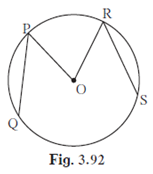(1) m(arc PR) = ∠POR = 70°

(2) ∠POQ + ∠ QOS + ∠ROS + ∠POR = 360°

As PQ = RS, ∠ POQ = ∠ROS = 80°

⇒ ∠POQ + ∠ QOS + ∠ROS + ∠POR = 360°

⇒ 80 + ∠QOS + 80 + ∠ 70 = 360

⇒ 230 + ∠ QOS = 360

⇒ ∠QOS = 130°

m(arc QS) = ∠QOS = 130°

(3) m(arc QSR) = ∠QOS + ∠ROS = 130 + 80 = 210°

Question 24.

In figure 3.93, m(arc WY) = 44°,m(arc ZX) = 68°, then

(1) Find the measure of ∠ ZTX.

(2) If WT = 4.8, TX = 8.0,YT = 6.4, find TZ.

(3) If WX = 25, YT = 8,YZ = 26, find WT.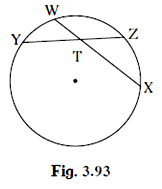(1)Given: m(arc WY) = 44°,m(arc ZX) = 68°

We know that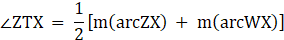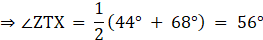(2)Given: WT = 4.8, TX = 8.0, YT = 6.4

We know that WT × TX = YT × TZ {Using secant-tangent theorem}

⇒ 6.4 × TZ = 4.8 × 8

⇒ TZ = 6

(3)Given: WX = 25, YT = 8,YZ = 26

Let WT = x and TX = 25-x

WT × TX = YT × TZ

⇒x(25-x) = 8 × 26

⇒ (x – 16)(x-9) = 0

⇒ WT = 16 or 9

Question 25.

In figure 3.94,

(1) m(arc CE) = 54°,

m(arc BD) = 23°, find measure of ∠CAE.

(2) If AB = 4.2, BC = 5.4,

(3) If AB = 3.6, AC = 9.0,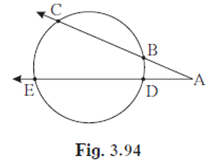(1)Given: m(arc CE) = 54°,

m(arc BD) = 23°

∠ CAE is an external angle.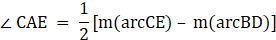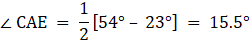(2)Given: AB = 4.2, BC = 5.4,AE = 12.0

Here, AB × AC = AD × EA

⇒ AD × 12 = 4.2 × 5.4

(3) Given AB = 3.6, AC = 9.0,

Here, AB × AC = AD × EA

⇒ AE × 5.4 = 3.6 × 9

⇒ AE = 6

Question 26.

In figure 3.95, chord EF || chord GH. Prove that, chord EG ≅ chord FH.

Fill in the blanks and write the proof.

Proof : Draw seg GF.

∠ EFG = ∠ FGH ...Alternate interior angles.......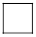(I)

∠ EFG = …………90° ………{inscribed angle theorem}(II)

∠ FGH = …………… 90° ………….{inscribed angle theorem} (III)

∴m(arc EG) = ……………90°…………. from (I), (II), (III).

chord EG ≅ chord FH ..........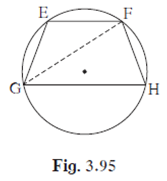Proof : Draw seg GF.

∠ EFG = ∠ FGH {Alternate interior angles} (I)

∠ EFG = 90°{inscribed angle theorem}(II)

∠ FGH = 90°{inscribed angle theorem} (III)

∴m(arc EG) = 90° from (I), (II), (III).

chord EG ≅ chord FH {Corresponding chords of congruent arcs of a circle (or congruent circles) are congruent}

Question 27.

In figure 3.96 P is the point of contact.

(1) If m(arc PR) = 140°,∠ POR = 36°,find m(arc PQ)

(2) If OP = 7.2, OQ = 3.2,find OR and QR

(3) If OP = 7.2, OR = 16.2,find QR.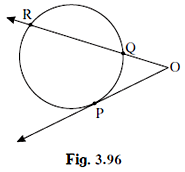(1)Given: m(arc PR) = 140°,∠ POR = 36°

∠ ROP is an external angle.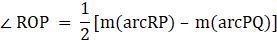⇒m(arc PQ) = 140° -2 × 36°

⇒m(arc PQ) = 68°

(2)Given: OP = 7.2, OQ = 3.2

Here, RO × OQ = OP2

⇒ RO × 3.2 = 7.2 × 7.2

⇒ RO = 16.2

QR = RO – OQ = 16.2 – 3.2 = 13

(3) Given: OP = 7.2, OR = 16.2

Here, RO × OQ = OP2

⇒ 16.2 × OQ = 7.2 × 7.2

⇒ OQ = 3.2

QR = RO – OQ = 16.2 – 3.2 = 13

Question 28.

In figure 3.97, circles with centres C and D touch internally at point E. D lies on the inner circle. Chord EB of the outer circle intersects inner circle at point A. Prove that, seg EA ≅seg AB.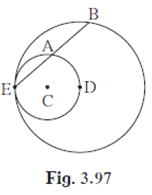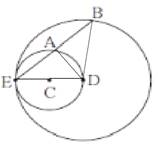We see that the line joining D to E passes through C.

In the smaller circle,

A lies in the semicircle,

⇒ DA is perpendicular on the chord EB of the bigger circle.

We know that perpendicular from the center bisects the chord.

Therefore, EA = AB.

Question 29.

In figure 3.98, seg AB is a diameter of a circle with centre O . The bisector of∠ ACB intersects the circle at point D. Prove that, seg AD ≅seg BD.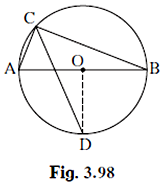Complete the following proof by filling in the blanks.

Proof: Draw seg OD.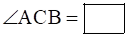…90° ............ angle inscribed in semicircle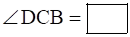........45° .... CD is the bisector of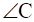m(arc DB) =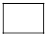........45° .. inscribed angle theorem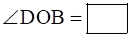…............90° definition of measure of an arc (I)

seg OA ≅seg OB .......radii of the circle...(II)

∴line OD isof seg AB ......bisector.... From (I) and (II)

Proof: Draw seg OD.

∠ ACB = 90° {angle inscribed in semicircle}

∠ DCB = 45° {CD is the bisector of}

m(arc DB) = 45° {inscribed angle theorem}

∠ DOB = 90° {definition of measure of an arc} (I)

seg OA ≅seg OB {radii of the circle}(II)

∴line OD is bisector of seg AB From (I) and (II)

Question 30.

In figure 3.99, seg MN is a chord of a circle with centre O. MN = 25,L is a point on chord MN such that ML = 9 and d(O,L) = 5.

Find the radius of the circle.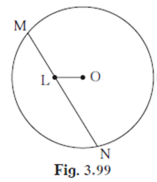The figure is shown below: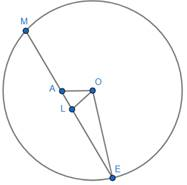Draw perpendicular on MN from the center O.

Mark the point as A. Join O to N.

As we know that perpendicular on a chord bisects the chord.

AM = MN/2

⇒ AM = 25/2 = 12.5

Given that LM = 9

⇒ LM + LA = AM

⇒ 9 + LA = 12.5

⇒ LA = 3.5

In Δ OAL,

⇒ OL2 = OA2 + AL2

⇒ 52 = OA2 + (3.5)2

⇒ OA2 = 25-12.25

⇒ OA2 = 12.75

In Δ OAN,

⇒ ON2 = OA2 + AN2

⇒ ON2 = 12.75 + (12.5)2

⇒ ON2 = 12.75 + 156.25

⇒ ON2 = 169

⇒ ON = 13

Therefore, the radius of the circle is 13.

Question 31.

In figure 3.100, two circles intersect each other at points S and R. Their common tangent PQ touches the circle at points P, Q.

Prove that, ∠ PRQ + ∠ PSQ = 180°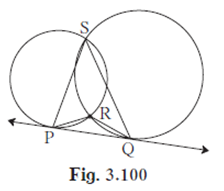We join R to S,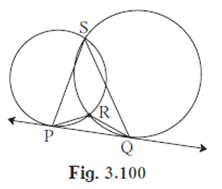As PQ is the tangent at P, we have

∠RPQ = ∠PSR …………..(1)

As PQ is tangent at Q, we have

∠RQP = ∠RSQ …………………(2)

In ΔRPQ, we have

⇒ ∠RPQ + ∠RQP + ∠ PRQ = 180° (Sum of all angles of a triangle)

⇒ ∠PSR + ∠RSQ + ∠PRQ = 180° (From (1) and (2))

⇒ ∠PSQ + ∠PRQ = 180° (∠PSR + ∠RSQ = ∠PSQ)

Hence Proved.

Question 32.

In figure 3.101, two circles intersect at points M and N. Secants drawn through M and N intersect the circles at points R, S and P, Q respectively.

Prove that :seg SQ || seg RP.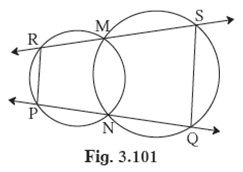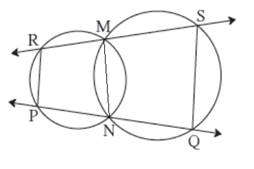We join MN.

As PRMN is a cyclic quadrilateral,

∠R + ∠PNM = 180° …………………..(1) (opposite angles of a cyclic quadrilateral)

Also, QSMN is a cyclic quadrilateral,

∠S + ∠ QNM = 180° ……………………(2) (opposite angles of a cyclic quadrilateral)

∠ R + ∠S + ∠ PNM + ∠QNM = 360°

⇒ ∠ R + ∠S + 180 = 360 (PQ is a straight line)

⇒ ∠ R + ∠S = 180°

Similarly we have,

As PRMN is a cyclic quadrilateral,

∠P + ∠RMN = 180° …………………..(3) (opposite angles of a cyclic quadrilateral)

Also, QSMN is a cyclic quadrilateral,

∠Q + ∠ SMN = 180° ……………………(4) (opposite angles of a cyclic quadrilateral)

∠ P + ∠Q + ∠ RMN + ∠SMN = 360°

⇒ ∠ P + ∠Q + 180 = 360 (RS is a straight line)

⇒ ∠ P + ∠Q = 180°

Therefore, PR ∥ SQ.

Question 33.

In figure 3.102, two circles intersect each other at points A and E. Their common secant through E intersects the circles at points B and D. The tangents of the circles at points Band D intersect each other at point C.

Prove thatABCD is cyclic.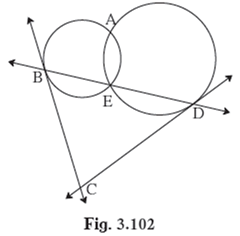We join A to B and A to D and A to E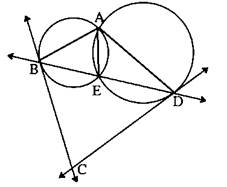As BC is a tangent at B, we have

∠ CBD = ∠BAE ………….(1)

As CD is a tangent at D, we have

∠ CDB = ∠DAE ………….(2)

In ΔBCD, we have

⇒ ∠CBD + ∠CDB + ∠ BCD = 180° (Sum of all angles of a triangle)

⇒ ∠BAE + ∠DAE + ∠BCD = 180° (From (1) and (2))

We have ∠A + ∠C = 180° (Proved above)

⇒ ∠A + ∠B + ∠C + ∠D = 360°

⇒ ∠B + ∠D + 180 = 360

⇒ ∠B + ∠D = 180

Therefore, opposite angles of the quadrilateral sum to 180. Hence ABCD is a cyclic quadrilateral.

Question 34.

In figure 3.103, seg ADside BC, seg BEside AC, seg CFside AB. Point O is the orthocentre. Prove that , point O is the incentre of∆ DEF.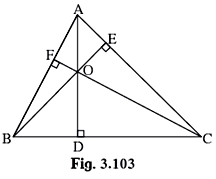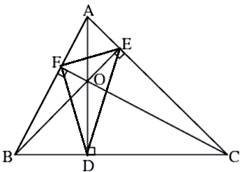Join D to E , D to F and E to F.

In ΔABE,

⇒ ∠ ABE + ∠BAE + ∠BEA = 180° (Sum of all angles of a triangle)

⇒ ∠ABE + ∠ BAE + 90 = 180

⇒ ∠ABE + ∠ BAE = 90

⇒ ∠ABO + ∠ BAC = 90

⇒ ∠ABO = 90 - ∠ BAC ………………….(1)

We have ∠F = 90 , ∠D = 90

⇒ ∠B + ∠F + ∠O + ∠D = 360°

⇒ ∠B + ∠O + 180 = 360

⇒ ∠B + ∠O = 180

Therefore, BFOD is a cyclic quadrilateral.

∠FBO = ∠FDO (angle by the same arc)

⇒ ∠ABO = ∠FDO

From (1),

⇒ ∠ FDO = 90 - ∠BAC ………………….(2)

In ΔAFC,

⇒ ∠ CAF + ∠FCA + ∠AFC = 180° (Sum of all angles of a triangle)

⇒ ∠CAF + ∠ FCA + 90 = 180

⇒ ∠CAF + ∠ FCA = 90

⇒ ∠BAC + ∠ OCE = 90

⇒ ∠OCE = 90 - ∠ BAC ………………….(3)

We have ∠E = 90 , ∠D = 90

⇒ ∠C + ∠E + ∠O + ∠D = 360°

⇒ ∠C + ∠O + 180 = 360

⇒ ∠C + ∠O = 180

Therefore, CEOD is a cyclic quadrilateral.

∠ODE = ∠OCE (angle by the same arc)

From (3),

⇒ ∠ ODE = 90 - ∠BAC …………(4)

From (2) and (4) we conclude,

∠FDO = ∠ODE

OD bisects ∠D.

Similarly, we can prove that OE bisects ∠E and OF bisects ∠F.

Hence O is the incenter of ΔDEF.